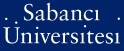# Compound invariants and mixed F-, DF-power spaces

Chalov, P. A. and Terzioğlu, Tosun and Zahariuta, V. P. (1998) Compound invariants and mixed F-, DF-power spaces. Canadian journal of mathematics, 50 (6). pp. 1138-1162. ISSN 0008-414XPDF - Repository staff only - Requires a PDF viewer such as GSview, Xpdf or Adobe Acrobat Reader171Kb

## Abstract

The problems on isomorphic classification and quasiequivalence of bases are studied for the class of mixed $F$-, $\DF$-power series spaces, {\it i.e.} the spaces of the following kind $$G(\la,a)=\lim_{p \to \infty} \proj \biggl(\lim_{q \to \infty}\ind \Bigl(\ell_1\bigl(a_i (p,q)\bigr)\Bigr)\biggr),$$ where $a_i (p,q)=\exp\bigl((p-\la_i q)a_i\bigr)$, $p,q \in \N$, and $\la =( \la_i)_{i \in \N}$, $a=(a_i)_{i \in \N}$ are some sequences of positive numbers. These spaces, up to isomorphisms, are basis subspaces of tensor products of power series spaces of $F$- and $\DF$-types, respectively. The $m$-rectangle characteristic $\mu_m^{\lambda,a}(\delta,\varepsilon; \tau,t)$, $m \in \N$ of the space $G(\la,a)$ is defined as the number of members of the sequence $(\la_i, a_i)_{i \in \N}$ which are contained in the union of $m$ rectangles $P_k = (\delta_k, \varepsilon_k] \times (\tau_k, t_k]$, $k = 1,2, \ldots, m$. It is shown that each $m$-rectangle characteristic is an invariant on the considered class under some proper definition of an equivalency relation. The main tool are new compound invariants, which combine some version of the classical approximative dimensions (Kolmogorov, Pe{\l}czynski) with appropriate geometrical and interpolational operations under neighborhoods of the origin (taken from a given basis).

Item Type: Article Q Science > QA Mathematics 11447 Tosun Terzioğlu 10 Apr 2009 16:49 25 May 2011 14:10

Repository Staff Only: item control page